# Test: Inequalities In A Triangle

## 15 Questions MCQ Test Mathematics (Maths) Class 9 | Test: Inequalities In A Triangle

Description
This mock test of Test: Inequalities In A Triangle for Class 9 helps you for every Class 9 entrance exam. This contains 15 Multiple Choice Questions for Class 9 Test: Inequalities In A Triangle (mcq) to study with solutions a complete question bank. The solved questions answers in this Test: Inequalities In A Triangle quiz give you a good mix of easy questions and tough questions. Class 9 students definitely take this Test: Inequalities In A Triangle exercise for a better result in the exam. You can find other Test: Inequalities In A Triangle extra questions, long questions & short questions for Class 9 on EduRev as well by searching above.
QUESTION: 1

Solution:
QUESTION: 2

Solution:
QUESTION: 3

### Which of the following does not represent the sides of a triangle?

Solution:
QUESTION: 4

In figure, which of the following statement is true?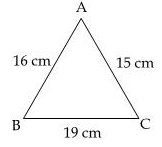Solution: Since, angle opposite to the smallest side is the smallest angle,<b (opposite to smallest side AC = 15cm) is the smallest angle.
QUESTION: 5

In triangle ABC, ∠B = 35°, ∠C = 65° and the bisector of ∠BAC meets BC in P, choose the correct order of inequality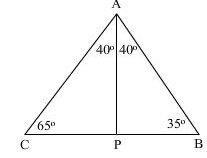Solution:
QUESTION: 6

If length of the largest side of a triangle is 12 cm then other two sides of triangle can be:​

Solution:
QUESTION: 7

Two sides of triangle are of lengths 5 cm and 1.5 cm. The length of the third side of the triangle cannot be​

Solution:
QUESTION: 8

In a triangle ABC this statement will always be true.

Solution:
QUESTION: 9

In ΔPQR, S is any point on the side QR, then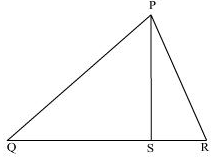Solution:
QUESTION: 10

In ΔABC, ∠A = 40° and ∠B = 60°. The longest side is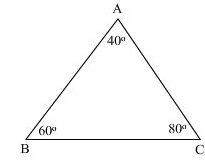Solution:
QUESTION: 11

The perimeter of a triangle is…. the sum of its medians.

Solution:
QUESTION: 12

It is not possible to construct a triangle when its sides are:​

Solution:
QUESTION: 13

Which of the following is incorrect ?

Solution:
QUESTION: 14

The figure shows that O is the centre of the circle and XOY is a diameter. If XZ is any other chord of the circle, then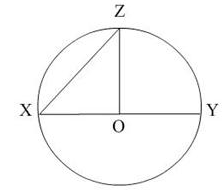Solution:
QUESTION: 15

In fig., which of the following statement is true?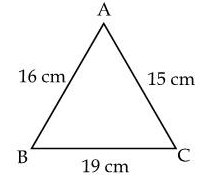Solution: